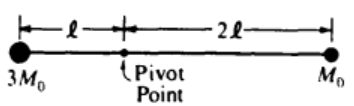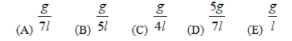# Problem: A light rigid rod with masses attached to its ends is pivoted about a horizontal axis as shown above. When released from rest in a horizontal orientation, the rod begins to rotate with an angular acceleration of magnitude

###### FREE Expert Solution

This problem involves net torque and acceleration.

Net torque:

$\overline{){\mathbit{\Sigma }}{\mathbit{\tau }}{\mathbf{=}}{\mathbit{I}}{\mathbit{\alpha }}}$ where I is the moment of inertia.

The torque due to Force:

$\overline{){\mathbit{\tau }}{\mathbf{=}}{\mathbit{r}}{\mathbit{F}}{\mathbf{sin}}{\mathbit{\theta }}}$

Moment of inertia of a point mass:

For a collection of point masses:

92% (126 ratings)###### Problem DetailsA light rigid rod with masses attached to its ends is pivoted about a horizontal axis as shown above. When released from rest in a horizontal orientation, the rod begins to rotate with an angular acceleration of magnitude